# Texas Go Math Grade 2 Lesson 1.7 Answer Key Different Ways to Show Numbers

Refer to our Texas Go Math Grade 2 Answer Key Pdf to score good marks in the exams. Test yourself by practicing the problems from Texas Go Math Grade 2 Lesson 1.7 Answer Key Different Ways to Show Numbers.

## Texas Go Math Grade 2 Lesson 1.7 Answer Key Different Ways to Show Numbers

Explore

Draw quick pictures to solve. Write how many tens and ones.
___________ tens ___________ ones

___________ tens ___________ ones

FOR THE TEACHER • Read this problem to children. Mrs. Peabody has 35 books on a cart to take to classrooms. She can use boxes that each hold 10 books and she can also place single books on the cart. What are two different ways she can put the books on the cart?
3 tens 5 one’s
0 tens 35 one’s
Explanation: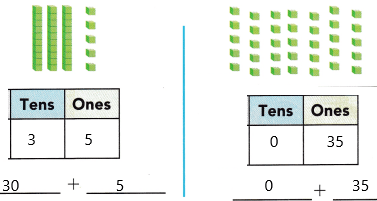First representation:
There are 3 tens of books which are equivalent to each block is 10
There are 5 ones of books which is equivalent to each block is 1
The total books in a cart: 30+5
3*10=30
5*1=5
Therefore, the total marble is 100+40+8.
the total:148
Second representation:
There are 0 ten of books which are equivalent to each block is 10
There are 35 ones of books which is equivalent to each block is 1
The total marbles are 0*10=0
35*1=35
Therefore, the total marble is 0+35
the total:35.

Math Talk
Mathematical Processes

Describe how you found different ways 35 books.
Place value in Maths describes the position or place of a digit in a number. Each digit has a place in a number. When we represent the number in general form the position of each digit will be expanded. Those positions start from a unit place or we also call it one’s position. The order of place value of digits of a number of right to left is units, tens, hundreds, thousands, ten thousand, a hundred thousand, and so on.
We placed digits in different ways in place values.

Model and Draw

Here are two ways to show 148.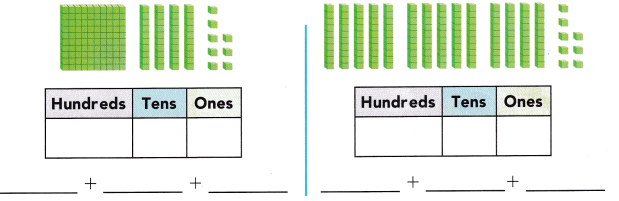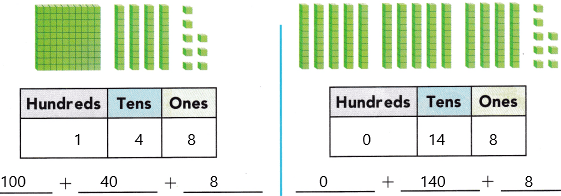Explanation:
First representation:
There is 1 block of hundreds which is equivalent to each block being 100.
There are 4 ten of blocks which are equivalent to each block is 10
There are 8 ones of block which is equivalent to each block is 1
The total marbles are 1*100=100
4*10=40
8*1=8
Therefore, the total marble is 100+40+8.
the total:148
Second representation:
There are 0 block of hundreds which is equivalent to each block being 100.
There are 14 ten of blocks which are equivalent to each block is 10
There are 8 ones of block which is equivalent to each block is 1
The total marbles are 0*100=0
14*10=140
8*1=8
Therefore, the total marble is 0+140+8
the total:148.

Share and Show

Write how many hundreds, tens, and ones are shown. Then use blocks. Draw and write another way to show the number.

Question 1.
213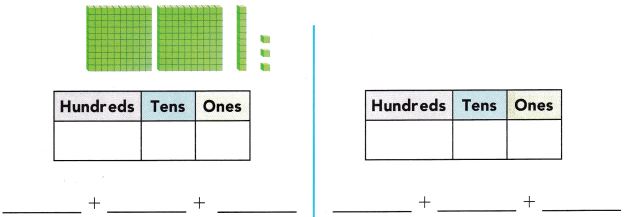Explanation:
First representation:
There is 2 block of hundreds which is equivalent to each block being 100.
There are 1 ten of blocks which are equivalent to each block is 10
There are 3 ones of block which is equivalent to each block is 1
The total marbles are 2*100=200
1*10=10
3*1=3
Therefore, the total marble is 200+10+3.
the total:213
Second representation:
There are 2 block of hundreds which is equivalent to each block being 100.
There are 0 ten of blocks which are equivalent to each block is 10
There are 13 ones of block which is equivalent to each block is 1
The total marbles are 2*100=200
0*10=0
13*1=13
Therefore, the total marble is 200+0+13.
the total:213.

Problem Solving

Question 2.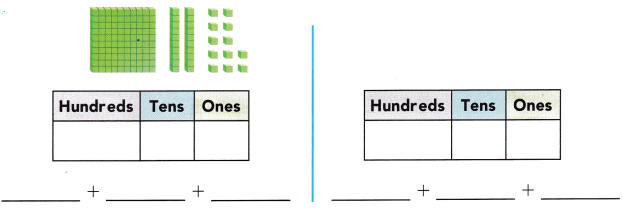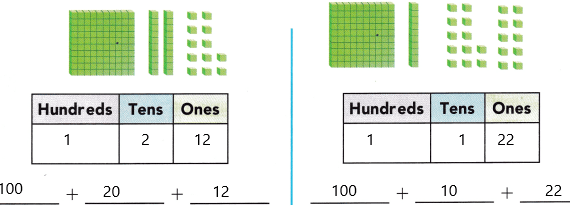Explanation:
First representation:
There is 1 block of hundreds which is equivalent to each block being 100.
There are 2 ten of blocks which are equivalent to each block is 10
There are 12 ones of block which is equivalent to each block is 1
The total marbles are 1*100=100
2*10=20
12*1=12
Therefore, the total marble is 100+20+12.
the total:.
Second representation:
There are 1 block of hundreds which is equivalent to each block being 100.
There are 1 ten of blocks which are equivalent to each block is 10
There are 22 ones of block which is equivalent to each block is 1
The total marbles are 1*100=100
1*10=10
22*1=22
Therefore, the total marble is 100+10+22.
the total:132.

Problem Solving

Write how many hundreds, tens, and ones are shown. Then use blocks. Draw and write another way to show the number.

Question 3.
144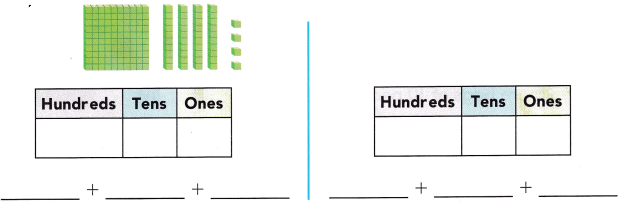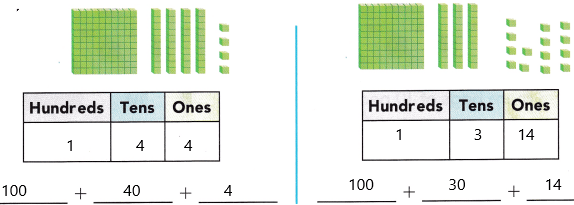First representation:
There is 1 block of hundreds which is equivalent to each block being 100.
There are 4 ten of blocks which are equivalent to each block is 10
There are 4 ones of block which is equivalent to each block is 1
The total marbles are 1*100=100
4*10=40
4*1=4
Therefore, the total marble is 100+40+4.
the total:144.
Second representation:
There are 1 block of hundreds which is equivalent to each block being 100.
There are 3 ten of blocks which are equivalent to each block is 10
There are 14 ones of block which is equivalent to each block is 1
The total marbles are 1*100=100
3*10=30
14*1=14
Therefore, the total marble is 100+30+14.
the total:144.

Marbles are sold in boxes, in bags, or as single marbles. Each box has 10 bags of marbles in it. Each bag has 10 marbles in it.Question 4.
H.O.T. Draw pictures to show two ways to buy 324 marbles.First representation: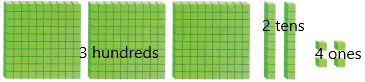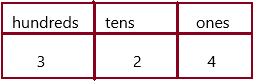There are 3 blocks of hundreds which is equivalent to each block being 100.
There are 2 ten of blocks which are equivalent to each block is 10
There are 4 ones of block which is equivalent to each block is 1
The total marbles are 3*100=300
2*10=20
4*1=4
Therefore, the total marbles 300+20+4.
the total:324.
second representation: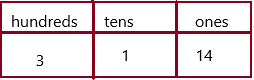There are 3 blocks of hundreds which is equivalent to each block being 100.
There are 1 ten of blocks which are equivalent to each block is 10
There are 14 ones of block which is equivalent to each block is 1
The total marbles are 3*100=300
1*10=10
14*1=14
Therefore, the total marbles 300+20+4.
the total:324.

Question 5.
H.O.T. Multi-Step There is only one box of marbles in the store. There are many bags of marbles and single marbles. Draw a picture to show a way to buy 312 marbles.There are 3 blocks of hundreds which is equivalent to each block being 100.
There are 1 ten of blocks which are equivalent to each block is 10
There are 2 ones of block which is equivalent to each block is 1
The total marbles are 3*100=300
1*10=10
1*2=2
Therefore, the total marbles 300+10+2=312.

Question 6.
Multi-Step Minnie has 220 pennies. She already filled these bags. How m more bags of 10 pennies can she fill?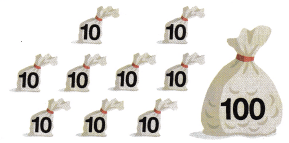(A) 2 more bags of 10 pennies
(B) 1 more bag of 10 pennies
(C) 3 more bags of 10 pennies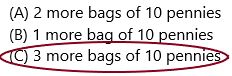3 more bags of 10 pennies means 3*10=30
Already one hundred bags are given and 9 tens bags are given
1*100=100
9*10=90
Total:100+90=190.
We need to find out the number of more bags needed to get 220 pennies. Assume it as X.
Subtract 220 and 190 then we get the needed bags.
X=220-190
X=30.
Therefore, this can be written as 3 more bags of 10 pennies are needed.
30=3*10.

Question 7.
Representations Write how many hundreds, tens, and ones are shown. Then use blocks. Draw and write another way to show the number.
238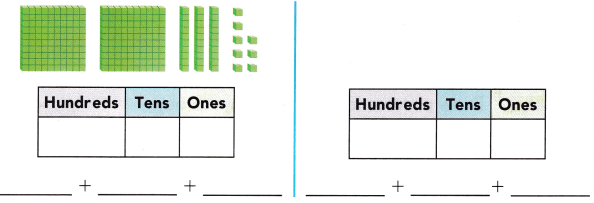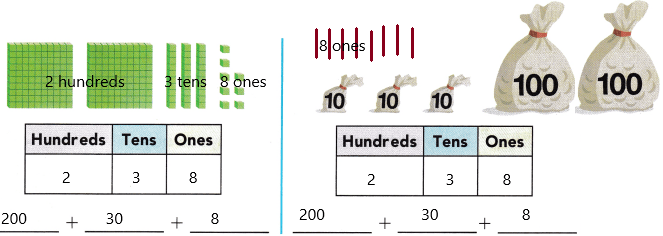Explanation:
Here we placed another representation having with 2 100’s and 3 10’s and then 8 1’s.
The place value of 238 is:
2 is in the hundreds place
3 is in the tens place
8 is in the one’s place
The word form of 238 is two hundred thirty-eight.

Question 8.
TEXAS Test Prep What number is shown with these blocks?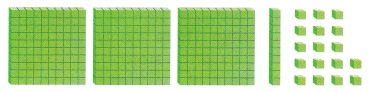(A) 317
(B) 342
(C) 327There are 3 blocks of hundreds which is equivalent to each block 100.
There is 1 block of tens which is equivalent to each block 10.
There are 17 ones that are equivalent to each block 1.
It was clearly representing that:
3 is in the hundreds place
1 in the tens place
17 is in the one’s place.
The total blocks:300+10+17
The total blocks:327.

TAKE HOME ACTIVITY • Write the number I56. Have your child draw quick pictures to show this number two ways.
Answer: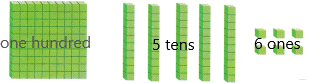We can write numbers in different ways such as expanded form, word form, standard form, pictorial representation, and place values.
Now 156 can be written in expanded form:
100+50+6.
The place value can be written as:
1 is in the hundreds place
5 is in the tens place
6 is in the one’s place.
The word form is one hundred fifty-six.

Scroll to Top